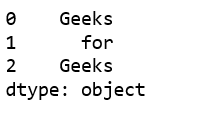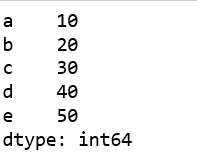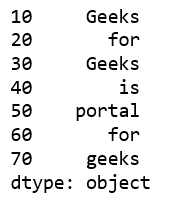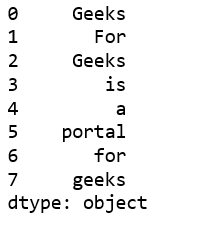# Creating a Pandas Series from Lists

• Difficulty Level : Medium
• Last Updated : 06 Jan, 2019

A `Series` is a one-dimensional labeled array capable of holding any data type (integers, strings, floating point numbers, Python objects, etc.). It has to be remembered that unlike Python lists, a Series will always contain data of the same type.

Let’s see how to create a Pandas Series from lists.

Attention geek! Strengthen your foundations with the Python Programming Foundation Course and learn the basics.

To begin with, your interview preparations Enhance your Data Structures concepts with the Python DS Course. And to begin with your Machine Learning Journey, join the Machine Learning - Basic Level Course

Method #1 : Using `Series()` method without any argument.

 `# import pandas as pd``import` `pandas as pd`` ` `# create Pandas Series with default index values``# default index ranges is from 0 to len(list) - 1``x ``=` `pd.Series([``'Geeks'``, ``'for'``, ``'Geeks'``])`` ` `# print the Series``print``(x)`

Output :Method #2 : Using `Series()` method with `'index'` argument.

 `# import pandas lib. as pd``import` `pandas as pd`` ` `# create Pandas Series with define indexes``x ``=` `pd.Series([``10``, ``20``, ``30``, ``40``, ``50``], index ``=``[``'a'``, ``'b'``, ``'c'``, ``'d'``, ``'e'``])`` ` `# print the Series``print``(x)`

Output :Another example:

 `# import pandas lib. as pd``import` `pandas as pd`` ` `ind ``=` `[``10``, ``20``, ``30``, ``40``, ``50``, ``60``, ``70``]`` ` `lst ``=` `[``'Geeks'``, ``'for'``, ``'Geeks'``, ``'is'``,``            ``'portal'``, ``'for'``, ``'geeks'``]``             ` `# create Pandas Series with define indexes``x ``=` `pd.Series(lst, index ``=` `ind)`` ` `# print the Series``print``(x)`

Output:Method #3: Using `Series()` method with multi-list

 `# importing pandas ``import` `pandas as pd`` ` `# multi-list``list` `=` `[ [``'Geeks'``], [``'For'``], [``'Geeks'``], [``'is'``],``         ``[``'a'``], [``'portal'``], [``'for'``], [``'geeks'``] ]``          ` `# create Pandas Series``df ``=` `pd.Series((i[``0``] ``for` `i ``in` `list``))`` ` `print``(df)`

Output:My Personal Notes arrow_drop_up